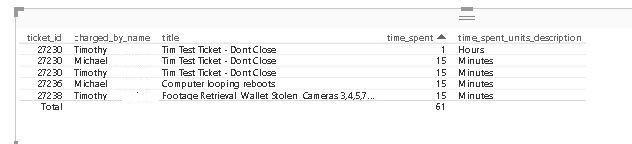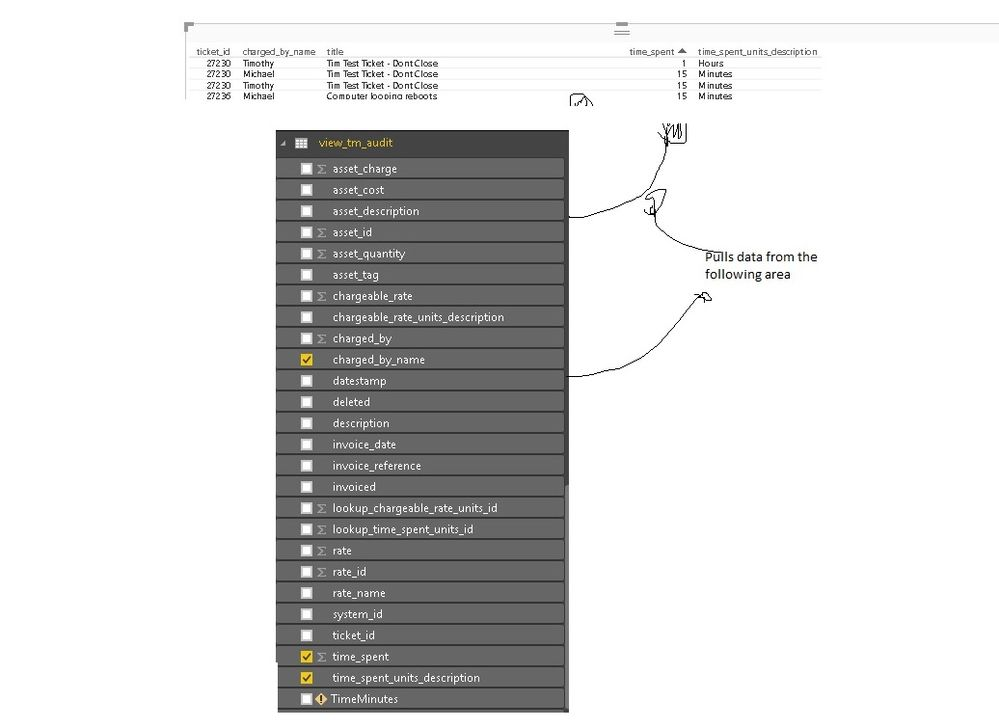cancel
Showing results for
Did you mean:Helper I

## Convert time to fraction (15mins to 0.25)

Hello All,

I am just experimenting with PowerBi and wanted to know if there was a way to convert time from a whole figure to a fraction?

Problem iam having is when its adding up times pBI is adding 15 mins and 1 hr to the same "tally"

I have tried messing around with IF statements and Modeling and just wondered if anyone would know if it is possible to have DAX add the total to look;

IF time_spent_description = Minutes (add them)

then have the total show as 2 Hrs

I have tried adding another column under Queries and working through other means, but because the data is being pulled from SQL directly it says i cant add or modify the QueriesThanks!

1 ACCEPTED SOLUTIONMicrosoft

@Wise1

In this scenario, I think you could add a numerator column based on the "time_spent_units_description" column with SWITCH() function.

`Numerator= SWITCH(Table[time_spent_units_description],"Hour",1,"Minute",60,"Second",60*60)`

Then you can just use the "time_spent" divide by Numerator.

`Time in Fraction = Table[time_spent]/Table[Numerator]`

Regards,

6 REPLIES 6Helper I

Hi @Wise1

One option is to convert everything into minutes with an IF-formula:

Create a new column:

Time minutes = if(Table1[units]="Hour";Table1[time_spent]*60;Table1[time_spent])

Another option is to create it into time using an IF formula:

Create a new column:

• Time 2 = If (Table1[units]="Hour";Table1[time_spent] & ":00:00"; "00:" & Table1[time_spent] & ":00")
• Convert it to time format
• Create a measure Total time 2 = Sum(Time 2)
• With this formule you obviously have the have a bit of control over how data are entered ( 120 minutes won' work well in this formula I think)

Hope this helps,

EspenHelper I

Hello,

Thanks for taking the time to reply to me, i will have a play around with the forumula you sent

Problem i have is because iam pulling this data with DirectQuery, and i cant add any columns (unless there is a way iam not aware of to do this!)Helper I

Hi @Wise1

There are two different "new columns".

1. In the query when you are shaping your data
2. In the Power BI desktop (where make alle you new measures and graphs)

In the formula in the previous post I've used the 2nd. Se creen shot:Regards,

EspenHelper I

Apologies for the delay in my reply, its been busy few days with xmas upon us

I have been messing around with a number of different ways to represent this based on the formula you let me know about, but I keep getting errors when i try to reference the table

ie:

Timeminutes = IF(;view_tm_audit[time_spent]*60;Table1[time_spent])Thanks for the tip of being able to add a column under the powerbi desktop!Microsoft

@Wise1

In this scenario, I think you could add a numerator column based on the "time_spent_units_description" column with SWITCH() function.

`Numerator= SWITCH(Table[time_spent_units_description],"Hour",1,"Minute",60,"Second",60*60)`

Then you can just use the "time_spent" divide by Numerator.

`Time in Fraction = Table[time_spent]/Table[Numerator]`

Regards,Helper I

thanks for explaining that! Sorry ive been enjoying the XMAS break so getting back into the swing of things for 2017

this works 🙂

You sir are a wizard